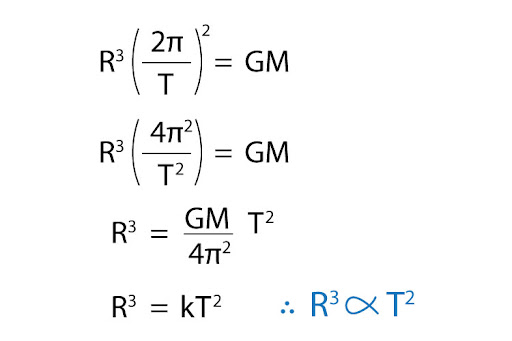# "How do I derive Kepler's 3rd law using Newton's Law of gravitation, in the case of a circular orbit?"

Before we dive into the derivation of Kepler’s Third Law from Newton’s Law of gravitation, we have to first understand what each of these laws mean. First off, we have Newton’s Law of Gravitation, which states that the gravitational force of attraction between two massive objects is directly proportional to the product of their masses, and inversely proportional to the square of their separation: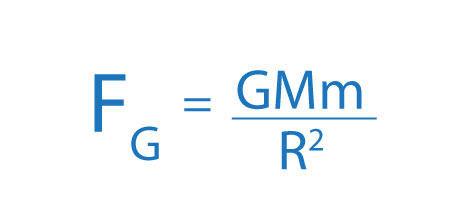Then we have Kepler’s Third Law of Planetary motion, which states that the square of an object’s orbital period is directly proportional to the cube of the semi-major axis of its orbit: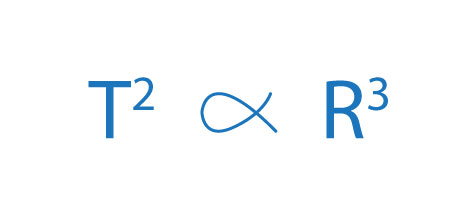Now, here we’re talking about a semi-major axis, because Kepler’s Third Law is based on a planet’s elliptical orbit, where there are two different axis: semi-major and semi-minor: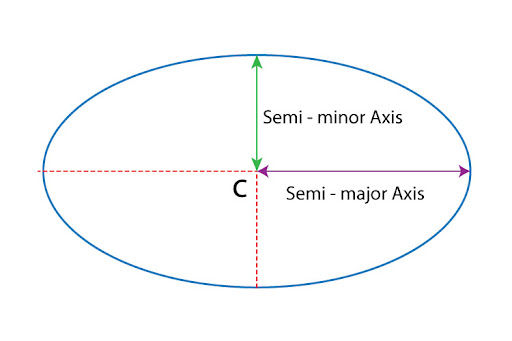However, when we’re dealing with a circular orbit, we realise that the semi-major and semi-minor axes become equal - that’s how we get the same radius throughout! In that case, we can draw a link between Kepler’s Third Law and Newton’s Law of Gravitation, where R is simply the radius of our circular orbit!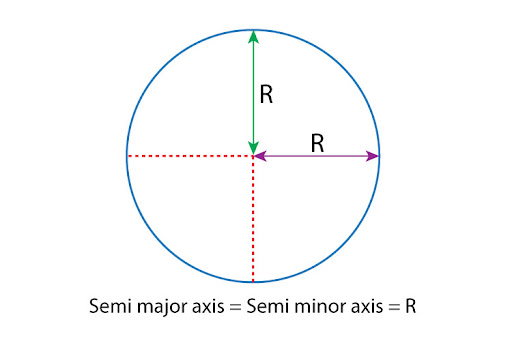Now, we can start our derivation by first acknowledging that a planet orbiting a mass (like the Sun!) can do so because the gravitational force is providing the centripetal force (Fc) needed to sustain this kind of circular motion: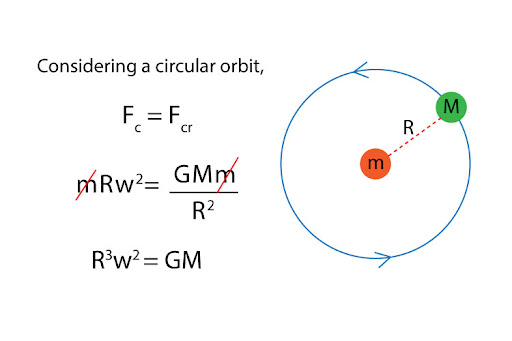Then, we must break down angular velocity further: since it is defined as the rate of change of angular displacement, we adjust this expression to apply to one complete revolution of the planet around the Sun. In that case, we get: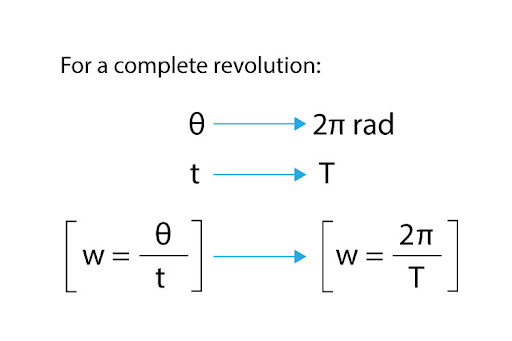All that’s left is some rearrangement, and we’re done!